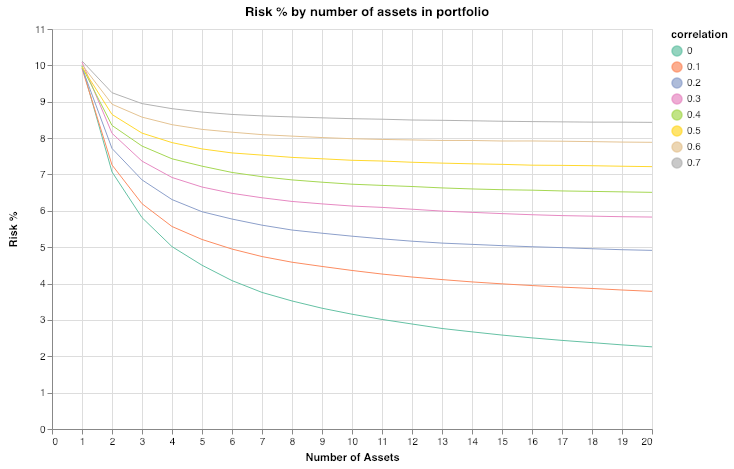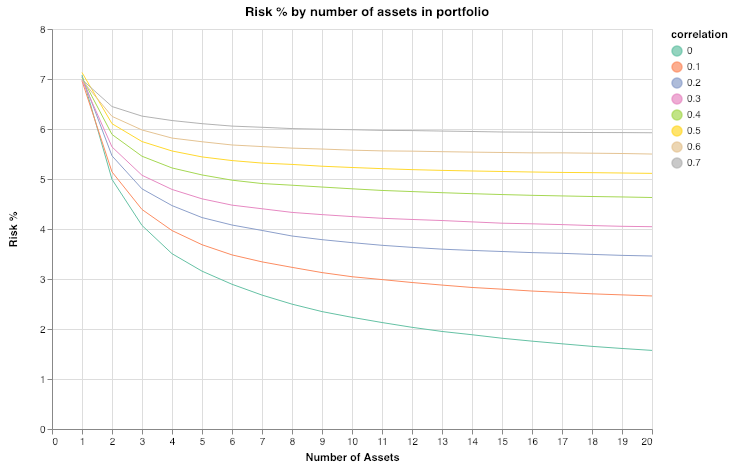# Ray Dalio's Holy Grail¶

### Reducing return/risk ratio through diversification.¶

https://www.investopedia.com/video/play/ray-dalio-his-portfolio-holy-grail/

From Principles: Life and Work by Ray Dalio.

From my earlier failures, I knew that no matter how confident I was in making any one bet I could still be wrong—and that proper diversification was the key to reducing risks without reducing returns. If I could build a portfolio filled with high-quality return streams that were properly diversified (they zigged and zagged in ways that balanced each other out), I could offer clients an overall portfolio return much more consistent and reliable than what they could get elsewhere.”

In this notebook, we'll explore what Ray Dalio referrs to as the Holy Grail of Investing, how increasing diversification we are able to reduce overall risk, as measured by the standard deviation of portfolio returns.
The idea is to show that, if we can find a basket of uncorrelated return streams (in practice we allow for low correlation), we can reduce the portfolio risk significantly by increasing the number of streams in our portfolio.

In :
%config InlineBackend.figure_format = "retina"

import numpy as np
import pandas as pd
import altair as alt

np.random.seed(42) # Set seed for reproducibility


We begin by creating a function that simulates n return streams with a given mean (mean) and standard deviation (risk), and a given average correlation (corr) between them.

In :
def correlated_streams(n, mean, risk, corr):
"""Generates n return streams with given average mean and risk,
and with an average correlation level corr.
"""
num_samples = 10_000
means = np.full(n, mean)

corr_mat = np.full((n, n), corr, dtype=np.dtype("d"))
np.fill_diagonal(corr_mat, 1,)
cov_mat = corr_mat * risk**2

streams = np.random.multivariate_normal(means, cov_mat, size=num_samples)

return streams.T


Just to make sure, let's do a sanity check.

In :
n = 5
mean, std, corr = 10, 15, 0.6

streams = correlated_streams(n, mean, std, corr)

In :
streams.mean(axis=1)

Out:
array([10.12229747,  9.92797016,  9.98877207, 10.05103342,  9.90978558])
In :
streams.std(axis=1)

Out:
array([15.07254044, 15.05168254, 15.17926238, 15.2192544 , 15.14908131])
In :
np.corrcoef(streams)

Out:
array([[1.        , 0.60676484, 0.61222918, 0.61179636, 0.60301561],
[0.60676484, 1.        , 0.61036834, 0.61049393, 0.61073826],
[0.61222918, 0.61036834, 1.        , 0.61526424, 0.61265281],
[0.61179636, 0.61049393, 0.61526424, 1.        , 0.605607  ],
[0.60301561, 0.61073826, 0.61265281, 0.605607  , 1.        ]])

This is the simplest way to construct such a portfolio. We make each pariwise correlation between assets equal to a given level corr. The important point is that the average of all the pairwise correlations should be equal to corr.

We'll create a helper function to calculate the pooled risk of a given number of return streams in the porfolio.

In :
def aggregate_risk(return_streams, n):
"""Returns the pooled risk (std) of the n first streams
in return_streams
"""
assert len(return_streams) >= n

aggregate_returns = np.sum(return_streams[:n], axis=0) / n
return aggregate_returns.std()


Next, we'll build our simulated dataset. We'll analyse return streams with risk levels in the range 1% - 14%, for varying number of streams ranging from 1 to 20.
We'll plot the risk levels for different average correlation, ranging from 0 to 0.7.

In :
max_assets = 20
assets = range(1, max_assets+1)

mean = 10 # Avg mean return of 10%
risk_levels = range(1, 15)

index = pd.MultiIndex.from_product([risk_levels, assets], names=["risk_level", "num_assets"])
simulated_data = pd.DataFrame(index=index)

for risk in risk_levels:
for corr in np.arange(0.0, .8, 0.1):
return_streams = correlated_streams(max_assets, mean, risk, corr)
risk_level = np.zeros(max_assets)
for num_assets in assets:
risk_level[num_assets-1] = aggregate_risk(return_streams, num_assets)
simulated_data.loc[(risk, ), round(corr, 1)] = risk_level

simulated_data.columns.names = ["correlation"]


Let's have a look at our dataset.

In :
simulated_data.query("risk_level == 14")

Out:
correlation 0.0 0.1 0.2 0.3 0.4 0.5 0.6 0.7
risk_level num_assets
14 1 14.139732 14.183844 14.062874 13.906914 14.016018 13.967769 14.047985 14.124923
2 9.971890 10.353876 10.942021 11.268121 11.719979 12.092035 12.523072 12.993926
3 8.109977 8.858116 9.628288 10.189106 10.866995 11.527557 11.936896 12.700021
4 6.986998 7.940064 9.000020 9.627738 10.427791 11.156455 11.632607 12.501330
5 6.264615 7.383155 8.476107 9.258889 10.160074 10.940836 11.467615 12.354408
6 5.716743 6.981279 8.180173 9.002885 9.964878 10.783878 11.360462 12.244618
7 5.295934 6.662273 7.948934 8.810349 9.824147 10.681967 11.263729 12.185342
8 4.947562 6.426823 7.746143 8.693509 9.722466 10.583549 11.178877 12.154764
9 4.661358 6.241620 7.579661 8.579598 9.654701 10.529631 11.145755 12.109616
10 4.442710 6.093881 7.460082 8.515337 9.593855 10.488526 11.119618 12.103459
11 4.239165 5.985589 7.368723 8.447905 9.551672 10.444280 11.096220 12.079866
12 4.067129 5.848384 7.261351 8.386694 9.511828 10.406193 11.076911 12.068993
13 3.899028 5.742075 7.186550 8.324049 9.473521 10.369270 11.052183 12.053648
14 3.748972 5.640994 7.124659 8.270063 9.439662 10.344146 11.026962 12.032337
15 3.621510 5.578849 7.071261 8.237998 9.407296 10.329517 11.021145 12.023096
16 3.504821 5.511867 7.032749 8.213322 9.375700 10.311569 11.012383 12.013513
17 3.388423 5.448855 6.989579 8.190652 9.355400 10.295327 11.004884 12.003186
18 3.286793 5.395965 6.953230 8.157502 9.333752 10.279044 10.988993 12.000082
19 3.202366 5.352398 6.913921 8.141816 9.315900 10.256781 10.980980 11.996158
20 3.127220 5.314700 6.896133 8.123222 9.304375 10.239964 10.973629 11.985602

We can already see how portfolio risk decreases as we add more assets, with sharper declines when we they have low correlation.

To recreate Dalio's chart (as seen in this video), we create a function that produces a plot given our simulated data and a risk level.

In :
def plot_risk_level(data, risk_level):
subset = data.query("risk_level == @risk_level")
stacked = subset.stack().reset_index(name="risk")

chart = alt.Chart(data=stacked)

highlight = alt.selection(type="single", on="mouseover",
fields=["correlation"], nearest=True)

base = chart.encode(
alt.X("num_assets", axis=alt.Axis(title="Number of Assets")),
alt.Y("risk", axis=alt.Axis(title="Risk %")),
alt.Color("correlation:N", scale=alt.Scale(scheme="set2")))

points = base.mark_circle().encode(
opacity=alt.value(0)
highlight
).properties(
height=400,
width=600,
title="Risk % by number of assets in portfolio"
)

lines = base.mark_line().encode(
size=alt.condition(~highlight, alt.value(1), alt.value(3)),
tooltip=["correlation"]
)

return points + lines


Let's see how diversification benefits a portfolio with assets that have a risk level of 10%.

In :
plot_risk_level(simulated_data, 10)

Out:A highly correlated portfolio does not benefit much from increased diversification. We get diminishing returns by adding highly correlated assets beyond 3 or 4.
In contrast, we can halve the risk by adding just 6 or 7 uncorrelated (or more realistically, weakly correlated) assets to a portfolio.

Let's plot the risk levels for a portfolio with returns streams with 7% risk.

In :
plot_risk_level(simulated_data, 7)

Out:## Conclusion¶

The benefits of diversification are generally well known: reduced risk through exposure to different sources of income.
The insight Dalio brings to the forefront, is that the construction of a diversified portfolio through a combination of uncorrelated return streams, significantly decreases our overall risk, raising in turn our return / risk ratio. By the careful mixing of uncorrelated assets, we capture true alpha, enabling us to use leverage to increase our returns.

In [ ]: